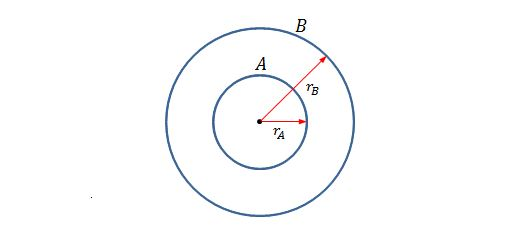Electricity and Magnetism

# Conductors

If the total charge on the surface of a conducting sphere is $2.3 \,\mu\text{C}$ and the diameter of the sphere is $1.1\text{ m},$ what is the magnitude of the electric field just outside the surface of the sphere, due to the surface charge?

The value of the permittivity constant is $\varepsilon_0=8.85 \times 10^{-12} \text{ C}^2\text{/N}\cdot\text{m}^2.$Suppose that a charged particle is held at the center of two concentric conducting spherical shells $A$ and $B,$ as shown in the above figure. The radius of sphere $A$ is $r_A=2\text{ cm}$ and that of sphere $B$ is $r_B=4\text{ cm}.$ The net flux $\Phi$ through a Gaussian sphere centered on the particle is \begin{aligned} -16.0 \times 10^5 \text{ N}\cdot\text{m}^2\text{/C} &\text{for } 0 < r < r_A \\ +8.0 \times 10^5 \text{ N}\cdot\text{m}^2\text{/C} &\text{for } r_A < r < r_B\\ -4.0 \times 10^5 \text{ N}\cdot\text{m}^2\text{/C} &\text{for } r > r_A, \end{aligned} where $r$ is the radius of the Gaussian sphere. Then what are the net charges of shell $A$ and shell $B,$ respectively?

The value of the permittivity constant is $\varepsilon_0=8.85 \times 10^{-12} \text{ C}^2\text{/N}\cdot\text{m}^2.$

If an isolated conductor has net charge $+15.0 \times 10^{-6}\text{ C}$ and a cavity with a point charge $q=+4.0 \times 10^{-6}\text{ C},$ what are the charge of the cavity wall $q_w$ and the charge on the outer surface $q_s?$

Consider a uniformly charged conducting sphere. If the radius of the sphere is $0.50\text{ m}$ and the surface charge density is $8.40 \,\mu\text{C/m}^2,$ what is the approximate total electric flux leaving the surface of the sphere?

The value of the permittivity constant is $\varepsilon_0=8.85 \times 10^{-12} \text{ C}^2\text{/N}\cdot\text{m}^2.$

If the magnitude of electric field just above the surface of a charged conducting cylinder is $2.8 \times 10^5 \text{ N/C},$ what is the surface charge density of the cylinder?

The value of the permittivity constant is $\varepsilon_0=8.85 \times 10^{-12} \text{ C}^2\text{/N}\cdot\text{m}^2.$

×